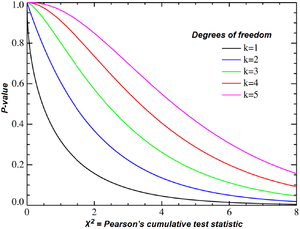# Chi-squared testChi-squared distribution, showing χ2 on the x-axis and P-value on the y-axis.

A chi-squared test, also written as χ2 test, is any statistical hypothesis test wherein the sampling distribution of the test statistic is a chi-squared distribution when the null hypothesis is true. Without other qualification, 'chi-squared test' often is used as short for Pearson's chi-squared test.

Chi-squared tests are often constructed from a sum of squared errors, or through the sample variance. Test statistics that follow a chi-squared distribution arise from an assumption of independent normally distributed data, which is valid in many cases due to the central limit theorem. A chi-squared test can be used to attempt rejection of the null hypothesis that the data are independent.

Also considered a chi-squared test is a test in which this is asymptotically true, meaning that the sampling distribution (if the null hypothesis is true) can be made to approximate a chi-squared distribution as closely as desired by making the sample size large enough. The chi-squared test is used to determine whether there is a significant difference between the expected frequencies and the observed frequencies in one or more categories.

## Examples of chi-squared tests with samples

One test statistic that follows a chi-squared distribution exactly is the test that the variance of a normally distributed population has a given value based on a sample variance. Such tests are uncommon in practice because the true variance of the population is usually unknown. However, there are several statistical tests where the chi-squared distribution is approximately valid:

### Pearson's chi-squared test

Pearson's chi-squared test, also known as the chi-squared goodness-of-fit test or chi-squared test for independence. It was developed in the year 1900. When the chi-squared test is mentioned without any modifiers or other precluding contexts, this test is often meant. For an exact test used in place of the chi-squared test, see Fisher's exact test.

### Yates's correction for continuity

Using the chi-squared distribution to interpret Pearson's chi-squared statistic requires one to assume that the discrete probability of observed binomial frequencies in the table can be approximated by the continuous chi-squared distribution. This assumption is not quite correct, and introduces some error.

To reduce the error in approximation, Frank Yates suggested a correction for continuity that adjusts the formula for Pearson's chi-squared test by subtracting 0.5 from the difference between each observed value and its expected value in a 2 × 2 contingency table. This reduces the chi-squared value obtained and thus increases its p-value.

## Chi-squared test for variance in a normal population

If a sample of size n is taken from a population having a normal distribution, then there is a result (see distribution of the sample variance) which allows a test to be made of whether the variance of the population has a pre-determined value. For example, a manufacturing process might have been in stable condition for a long period, allowing a value for the variance to be determined essentially without error. Suppose that a variant of the process is being tested, giving rise to a small sample of n product items whose variation is to be tested. The test statistic T in this instance could be set to be the sum of squares about the sample mean, divided by the nominal value for the variance (i.e. the value to be tested as holding). Then T has a chi-squared distribution with n − 1 degrees of freedom. For example, if the sample size is 21, the acceptance region for T with a significance level of 5% is between 9.59 and 34.17.

## Example chi-squared test for categorical data

Suppose there is a city of 1 million residents with four neighborhoods: A, B, C, and D. A random sample of 650 residents of the city is taken and their occupation is recorded as "white collar", "blue collar", or "no collar". The null hypothesis is that each person's neighborhood of residence is independent of the person's occupational classification. The data are tabulated as:

A B C D total
White collar 90 60 104 95 349
Blue collar 30 50 51 20 151
No collar 30 40 45 35 150
Total 150 150 200 150 650

Let us take the sample living in neighborhood A, 150, to estimate what proportion of the whole 1 million people live in neighborhood A. Similarly we take 349/650 to estimate what proportion of the 1 million people are white-collar workers. By the assumption of independence under the hypothesis we should "expect" the number of white-collar workers in neighborhood A to beThen in that "cell" of the table, we haveThe sum of these quantities over all of the cells is the test statistic. Under the null hypothesis, it has approximately a chi-squared distribution whose number of degrees of freedom areIf the test statistic is improbably large according to that chi-squared distribution, then one rejects the null hypothesis of independence.

A related issue is a test of homogeneity. Suppose that instead of giving every resident of each of the four neighborhoods an equal chance of inclusion in the sample, we decide in advance how many residents of each neighborhood to include. Then each resident has the same chance of being chosen as do all residents of the same neighborhood, but residents of different neighborhoods would have different probabilities of being chosen if the four sample sizes are not proportional to the populations of the four neighborhoods. In such a case, we would be testing "homogeneity" rather than "independence". The question is whether the proportions of blue-collar, white-collar, and no-collar workers in the four neighborhoods are the same. However, the test is done in the same way.

## Applications

In cryptanalysis, chi-squared test is used to compare the distribution of plaintext and (possibly) decrypted ciphertext. The lowest value of the test means that the decryption was successful with high probability. This method can be generalized for solving modern cryptographic problems.